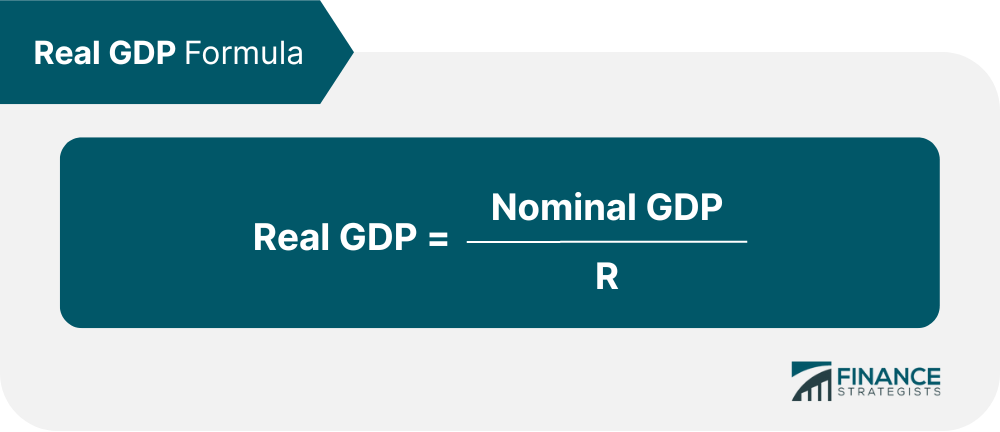# Real Gross Domestic Product (GDP)### Written byTrue Tamplin, BSc, CEPF® | Reviewed by Editorial Team

Updated on January 04, 2023

## Define Real GDP in Simple Terms

Real gross domestic product, or real GDP, is a measure of the economic output of a nation, adjusted for changes in the price of goods. Nominal GDP, often just called "GDP", measures the economic output of a nation using only current prices.

This makes calculation simple, and is effective when looking at a single year. However, when comparing GDP changes over the span of several years, the effects of inflation can distort the accuracy of the figure.

Real GDP rectifies this problem by adjusting current prices to match the prices of a base year in order to determine precisely how much of the change in GDP is due to changes in production volume and how much is due to changes in price.

## Calculating Real GDP

GDP is calculated using the following equation:R is a so-called GDP deflator. For example, if prices have increased by 1% since the base year, then R would be 1.01. If prices increased by 50%, then R would be 1.5.

## Real GDP Example

For example, let's say that Country A (for sake of simplicity, assume they only produce one commodity, such as rubber bands) had a nominal GDP of \$50 billion in 2000. In 2001, it had a nominal GDP of \$75 billion.

If only nominal GDP is considered, this would seem to be a substantial increase. However, the price of rubber bands also increased from \$1 per hundred in 2000 to \$1.50 per hundred in 2001. The real GDP would be \$75 billion / 1.5, or \$50 billion.

Unfortunately, Country A's increase in GDP can be attributed solely to an increase in price, and not to improvements in production.

## Real Gross Domestic Product (Real GDP)  FAQs

### What does real GDP stand for?

Real GDP stands for real gross domestic product.

### What is Real Gross Domestic Product?

Real gross domestic product, or real GDP, is a measure of the economic output of a nation, adjusted for changes in the price of goods.

### How do I calculate real GDP?

The formula for calculating real GDP is: Nominal GDP / R (where R is a so-called GDP deflator). For example, if prices have increased by 1% since the base year, then R would be 1.01. If prices increased by 50%, then R would be 1.5.

### How is GDP affected over the span of years?

When comparing GDP changes over the span of several years, the effects of inflation can distort the accuracy of the figure. Real GDP rectifies this problem by adjusting current prices to match the prices of a base year in order to determine precisely how much of the change in GDP is due to changes in production volume and how much is due to changes in price.

### What are the different types of GDP?

The different types of GDP measurements are Nominal GDP, GDP per capita, real GDP, and GDP growth rate.